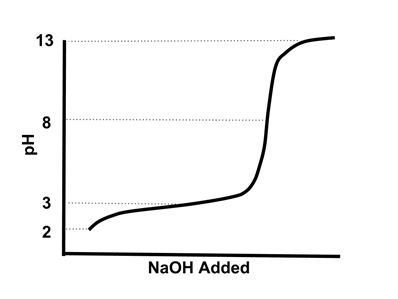# Solubility and Acid-Base Equilibrium

This quiz is based on AP Chemistry Enduring understanding: "Chemical equilibrium plays an important role in acid-base chemistry and in solubility." In particular it focuses on pH calculations, titrations, buffers, and solubility calculation problems.

Start Quiz

A solution has a hydroxide concentration of 2.0 x 10^-5. What is the pH of the solution?

1.5

9.3

7.3

13.0

Which pair of 1.0M solutions would have nearly the same concentration of hydronium ions?

HCl and HNO2

HF and HBr

HCl and HBr

HCl and HF

The diagram shows a titration curve of a weak acid with a strong base. What is the pKa of the weak acid?0

2

3

7

A 0.1 mol/L solution of formic acid (40 mL) is titrated with 0.20 mol sodium hydroxide (10 mL). What is the pH of the resulting solution? The pKa of formic acid is 3.77.

3.77

3.90

7

10.23

A chemist is working with a liquid substance that tastes bitter, feels slippery, and conducts an electric current. Which of the following is the chemist most likely working with?

A salt

A base

An acid

Distilled water

Complete the sentence.
A substance that is more basic has a _____ pH than a substance that is acidic.

Same

Lower

Higher

None of these

A pH of 3 is how many times more acidic than a pH of 5?

5

100

1000

Can’t say

What is the pH of 25 mL of a 0.1M FCH2COOH (fluoroacetic acid) solution titrated to its equivalence point with 25 mL of a 0.1M NaOH? pKa of fluoroacetic acid=2.60

6.98

7.65

10.87

11.40

Quiz/Test Summary
Title: Solubility and Acid-Base Equilibrium
Questions: 8
Contributed by: